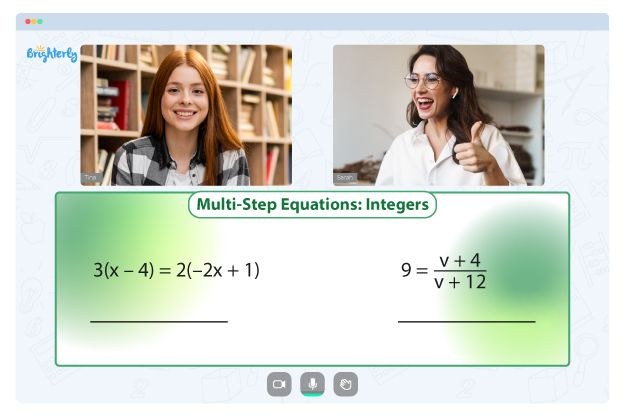# Negative Exponents Worksheets

The negative exponent rule states that any natural number raised to the power of a negative number will be divided using the number raised to the positive power of the same number. You can use a negative exponents worksheet to teach your kids about the workings of negative exponents.

The formula for the negative exponent is a−m = 1/am

Your kids need to understand and remember this formula for a negative exponent so that they can solve problems related to the math concept. You can use the negative exponent worksheet to help your child remember the rules using repeated teaching of the math concepts.

## Benefits of negative exponents worksheet PDF

Here are some of the benefits of the negative exponents practice worksheet:

### It helps parents and teachers make better decisions regarding their child’s studies

When you use exponents with negative base worksheets to study with your kids, you learn much more about exponents. In addition, some negative exponents worksheets come with answers at the back to show kids where they did wrong.

Math for Kids

Is Your Child Struggling With Math?
1:1 Online Math Tutoring### It helps students familiarize themselves with the negative exponent concept

The negative exponent may confuse kids if they learn about it only in class but have no study time at home. Using the exponents worksheets at home helps students familiarize themselves with the concept well enough to tackle related tasks that will be encountered later in problems.

You can download negative exponent worksheets with answer keys at the back. Use these worksheets to set classwork, assignments, and tests for the kids in your class to sharpen their knowledge of negative exponents.### Exponents with Negative Bases Worksheet PDF

Exponents with Negative Bases Worksheet### Negative Exponent Worksheet PDF

Negative Exponent Worksheet### Negative Exponents Practice Worksheet PDF

Negative Exponents Practice Worksheet### Negative Exponents Worksheet PDF

Negative Exponents Worksheet PDF

Struggling with Equations?• Start lessons with an online tutor.

Is your child finding it challenging to understand the concept of equations? An online tutor could be the solution.